﻿ Teaching Nano-Thermodynamics: Gibbs Energy of Single-Component NanoparticlesPublications are Open
Access in this journal
Article Versions
Export Article
• Normal Style
• MLA Style
• APA Style
• Chicago Style
Research Article
Open Access Peer-reviewed

### Teaching Nano-Thermodynamics: Gibbs Energy of Single-Component Nanoparticles

Jindřich Leitner , David Sedmidubský
World Journal of Chemical Education. 2017, 5(6), 206-209. DOI: 10.12691/wjce-5-6-4
Published online: January 05, 2018

### Abstract

Much attention has been paid to thermodynamic modeling of nanosystems. A common approach consists in addition of a surface/interface term to the Gibbs energy of bulk materials and application of general conditions of equilibrium. Some discrepancy still remains dealing with the expression for surface contribution to molar Gibbs energy and chemical potential of components. It is shown, that due to the nonextensive nature of the surface area, these contributions are different for molar and partial molar quantities. The consistent expressions for the molar Gibbs energy and chemical potential of a single-component spherical nanoparticle are put forward along with the simple derivation of the Kelvin and Gibbs-Thomson equations.

### 1. Introduction

Recently, much attention has been paid to calculation of phase diagrams of nanoalloys 1, 2, 3, 4, 5 as well as calculation of equilibrium constants of chemical reactions in nanosystems 6, 7, 8, 9. A common approach consists in addition of a surface/interface term to the Gibbs energy of bulk materials and application of general conditions of equilibrium in a closed system at constant temperature and pressure, namely equality of chemical potentials of individual components in coexisting phases or zero Gibbs energy of reaction. Surprisingly, some discrepancy still remains dealing with the expression of chemical potential of the involved components. Two quite similar but diverse terms are used for the surface contribution to Gibbs energy of spherical nanoparticles of radius r, namely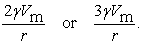This apparent controversy has been a subject of discussion and has been referred in a number of recently published papers [10-15] 10. The purpose of our contribution is to correctly derive relations for the molar Gibbs energy and chemical potential of single-component spherical nanoparticle and explain this apparent discrepancy. Moreover, the relation for chemical potential of nanoparticle is used for simple elucidation of the Kelvin and Gibbs-Thomson equations.

### 2. Homogeneous Functions

First, we recall the fundamental theorems abouthomogeneous functions for a better understanding of further explanation. A function F(x) of variable x is called the homogeneous function of the k-degree if it holds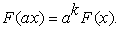(1)

The Euler theorem gives for any homogeneous function of the k-degree the relation 16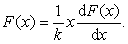(2)

For the homogeneous functions of the first order, and only for them, the relationship (2) results in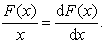(3)

### 3. Gibbs Energy of Single-component Nanoparticles

3.1. Total Gibbs Energy of Nanoparticle

Suppose a spherical nanoparticle of radius r consisting of n moles of the given substance. The total Gibbs energy of this particle Gnp can be express as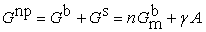(4)

The superscripts b and s stand for the bulk and surface terms, respectively, Gbm is the molar Gibbs energy of the bulk, γ denotes the surface energy (a reversible work that must be exerted to create a new surface/interface of unit area) and A is the total surface/interface area. The bulk contribution Gb is a homogeneous function of the first order of the amount n (extensive properties) 16 and it holds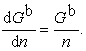(5)

The surface contribution to the Gibbs energy of a spherical nanoparticle of radius r can be expressed as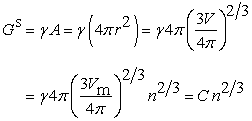(6)

where C = γ(4π)1/3(3Vm)2/3 and Vm stands for molar volume. It follows from eq. (6) that the surface contribution to Gibbs energy is not an extensive property 17, 18, 19 but a homogeneous function of the order 2/3 (degree of homogeneity k = 2/3) of the amount n. Hence it yields, according to the Euler theorem (2), the relation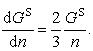(7)

The total Gibbs energy of the nanoparticle is not a homogeneous function of the amount n as the condition (1) is not met.

3.2. Molar Gibbs Energy of Nanoparticle

The molar Gibbs energy of a spherical single-component nanoparticle of radius r is given as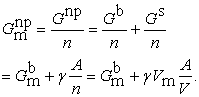(8)

Based on the simple geometrical relations valid for a spherical nanoparticle of radius r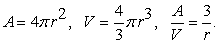(9)

the expression for the molar Gibbs energy is given as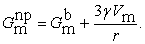(10)
3.3. Partial Molar Gibbs Energy (Chemical Potential) of Nanoparticle

The chemical potential (partial molar Gibbs energy) of a spherical single-component nanoparticle of radius r is given as a partial derivative of Gibbs energy G with respect to substance amount n at constant temperature and pressure. It holds for the bulk contributionNote 1.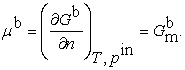(11)

Similarly the surface contribution can be expressed as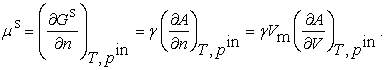(12)

We consider here only isomorphic changes of a particle, e.g. the changes of particle volume without any shape changes, and so (∂A/∂V) = 2/r.

Thus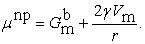(13)

The difference between the surface contribution to the molar Gibbs energy and chemical potential of Au nanoparticle of radius r is shown in Figure 1.

• Figure 1. (Color online) The difference between surface contribution to molar Gibbs energy and chemical potential of Au nanoparticle of radius r (Vm = 10.610-6 m3 mol-1, γ = 1.764 J m-2)

It follows from the above presented equations that both surface terms are correct but with a different meaning. Due to the fact, that equilibrium conditions are always derived from differential relations dG = 0 or dF = 0, the surface term with the multiplier 2 (eq. (13)) should be correctly used.

### 4. Applications for Gas-liquid and Solid-liquid Equilibria

Suppose a solid or liquid spherical nanoparticle (phase α) of radius r which is surrounded by a fluid phase (phase β). Let such a system be closed at a constant temperature T and pressure of fluid phase p. The pressure pin within the condensed nanoparticle is, due to the surface stress, higher than the pressure of fluid phase. If the pressure dependence of the condensed phase molar volume is neglected the following relation should be satisfied at equilibrium 20, 21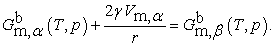(14)

This equation has the general form F(T,p,r) = 0 and setting one of the variables T, p or r, a dependence between the other two can be obtained.

Considering a liquid nanoparticle (nanodroplet) - vapor equilibrium at a constant temperature T and pressure pr the well-known Kelvin equation should be simply derived from Eq. (14)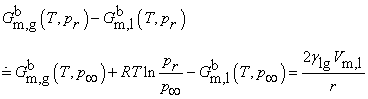(15)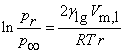(16)

where p is the equilibrium pressure of the vapor above the plane surface and pr above the surface with curvature 1/r. Note that the difference Gm,l(pr) - Gm,l(p) has been omitted. Calculated vapor pressures of selected liquid metals at their melting points above spherical nanodroplets of radius r according to the Kelvin equation (16) are shown in Figure 2.

• Figure 2. (Color online) Calculated vapor pressures of selected liquid metals at their melting points above spherical nanodroplets of radius r according to the Kelvin equation (16)

Considering a solid nanoparticle - liquid phase (melt) equilibrium at a constant temperature Tfus,r and pressure p, the Gibbs-Thomson equation should be derived in the form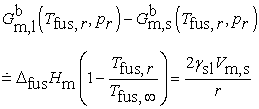(17)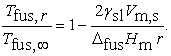(18)

Tfus,∞ is the equilibrium melting temperature for a macroscopic solid and Tfus,r for a spherical nanoparticle of radius r. Note that the temperature dependence of the enthalpy of melting has been omitted. Calculated melting temperatures of selected metals in the form of spherical nanoparticles of radius r according to the Gibbs-Thomson equation (18) are shown in Figure 3.

• Figure 3. (Color online) Calculated melting temperatures of selected metals in the form of spherical nanoparticles of radius r according to the Gibbs-Thomson equation (18)

### 5. Conclusion

In this article, a controversial issue in nano–thermodynamics, namely the correct form of surface contributions to the molar Gibbs energy and chemical potential of single–component nanoparticles is explained. To better understand this difference it is convenient to use a formal approach based on the homogeneous functions formalism. Moreover, using the correct form of the equilibrium condition (the equality of chemical potentials) simple derivation of Kelvin and Gibbs–Thomson equations is presented.

### Acknowledgements

This work was supported by Czech Science Foundation, grant No. 17-13161S.

### Note

1Constant pressure pin within a particle is considered here which is, due to the surface stress f, higher than the pressure p in the surroundings. The condition of constant pin means such a variation of the surroundings pressure p to eliminate any changes in pin associated with changes ot the particle radius r. These variables are interconnected via the Young-Laplace equation pin - p = 2f/r.

### References

  Guisbiers, G.; Mejia-Rosales, S.; Khanal, S.; Ruiz-Zepeda, F.; Whetten, R. L.; José-Yacaman, M. Gold-Copper Nano-Alloy, “Tumbaga”, in the Era of Nano: Phase Diagram and Segregation. Nano Lett. 14. 6718-6726. 2014. In article  Sim, K.; Lee, J. Phase stability of Ag-Sn alloy nanoparticles. J. Alloys Compounds 590. 140-146. 2014. In article View Article  Sopoušek, J.; Vřešťál, J.; Pinkas, J.; Brož, P.; Buršík, J.; Stýskalík, A.; Škoda, D.; Zobač, O.; Lee, J. Cu-Ni nanoalloy phase diagram - Prediction and experiment. CALPHAD 45. 33-39. 2014. In article View Article  Kroupa, A.; Káňa, T.; Buršík, J.; Zemanová, A.; Šob, M. Modelling of phase diagrams of nanoalloys with complex metallic phases: application to Ni-Sn. Phys. Chem. Chem. Phys. 17. 28200-28210. 2015. In article View Article  PubMed  Ghasemi, M.; Zanolli, Z.; Stankovski, M.; Johansson, J. Size- and shape-dependent phase diagram of In-Sb nano-alloys. Nanoscale 7. 17387-17396. 2015. In article View Article  PubMed  Du, J.; Zhao, R.; Xue, Y. Effects of sizes of nano-copper oxide on the equlibrium constant and thermodynamic properties for the reaction in nanosystem. J. Chem. Thermodynamics 45. 48-52. 2012. In article View Article  Du, J.; Zhao, R.; Xue, Y. Thermodynamic properties and equilibrium constant of chemical reaction in nanosystem: An theoretical and experimental study. J. Chem. Thermodynamics 55. 218-224. 2012. In article View Article  Cui, Z.; Duan, H.; Li, W.; Xue, Y. Theoretical and experimental study: the size dependence of decomposition thermodynamics of nanomaterials. J. Nanopart. Res. 17. 321 (11 pp.). 2015. In article  Li, W.; Cui, Z.; Duan, H.; Xue, Y. Effect of Nanoparticle size on the thermal decomposition thermodynamics in theory and experiment. Appl. Phys. A 122. 99 (12 pp.). 2016. In article  Kaptay, G. The Gibbs Equation versus the Kelvin and the Gibbs-Thomson Equations to Describe Nucleation and Equilibrium of Nano-Materials. J. Nanosci. Nanotechnol. 12. 2625-2633. 2012. In article View Article  PubMed  Kaptay, G. Nano-Calphad: extension of the Calphad method to systems with nano-phases and complexions. J. Mater. Sci. 47. 8320-8335. 2012. In article View Article  Makkonen, L. Comments on “The Gibbs Equation versus the Kelvin and the Gibbs-Thomson Equations to Describe Nucleation and Equilibrium of Nano-Materials”. Adv. Sci. Focus 1. 367-368. 2013. In article View Article  Lee, J.; Sim, K.J., General equations of CALPHAD-type thermodynamic description for metallic nanoparticle systems. CALPHAD 44. 129-132. 2014. In article View Article  Guenther, G.; Guillon, O. Models of size-dependent nanoparticle melting tested on gold. J. Mater. Sci. 49. 7915-7932. 2014. In article View Article  Bajaj, S.; Haverty, M. G.; Arróyave, R.; Goddard III FRSC, W. A.; Shankare, S. Phase stability in nanoscale material systems: extension from bulk phase diagrams Nanoscale, 7. 9868-9877. 2015. In article View Article  Blinder, S.M. Mathematical method in elementary thermodynamics. J. Chem. Education 43. 85-92. 1966. In article View Article  Letellier, P.; Mayaffre, A.; Turmine, M. Nonextensive approach to thermodynamics: Analysis and suggestion, and application to chemical reactivity. J. Phys. Chem. B 108. 18980-18987. 2004. In article View Article  Letellier, P.; Mayaffre, A.; Turmine, M. Solubility of nanoparticles: nonextensive thermodynamics approach J. Phys.: Condens. Matter 19. 436229 (9 pp). 2007. In article  Letellier, P.; Mayaffre, A.; Turmine, M. Melting point depression of nanosolids: Nonextensive thermodynamics approach. Phys. Rev. B 76. 045428 (8 pp). 2007. In article  Cammarata, R.C. Generalized thermodynamics of surfaces with applications to small solid systems. in Solid State Physics. 61. 1-75. 2009. In article View Article  Leitner, J.; Sedmidubský, D. Thermodynamic equilibria in system with nanoparticles. in Thermal Physics and Thermal Analysis. Springer International Publishing, 2017. 385-402. In article View ArticleThis work is licensed under a Creative Commons Attribution 4.0 International License. To view a copy of this license, visit http://creativecommons.org/licenses/by/4.0/